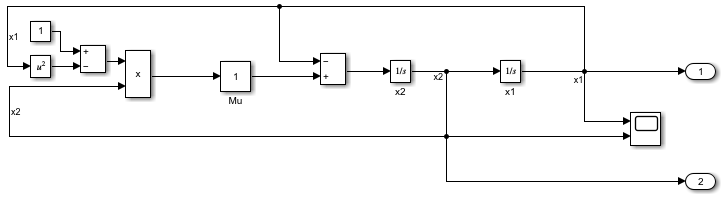# Max step size

Maximum step size for variable-step solver

Model Configuration Pane: Solver

## Description

Specify the largest time step, in seconds, for the variable-step solver to take in simulation.

## Dependencies

To enable this parameter, set the solver Type to `Variable-step`.

## Settings

`auto` (default) | scalar

By default, the Max step size value is `auto`, which indicates that the solver determines the maximum step size to use in the simulation. The way the solver determines the maximum step size depends on the type of variable-step solver selected.

• When the Solver parameter value is `discrete (no continuous states)`, the solver uses the smallest sample time in the model as the maximum step size.

• When the Solver parameter value is something other than `discrete (no continuous states)`, the maximum step size is determined based on the specified Start time and Stop time.

• When the stop time is `inf` or is equal to the start time, the maximum step size is `0.2`.

• For all other stop time values, the maximum step size is chosen such that the simulation has at least 50 time hits.

`${h}_{\mathrm{max}}=\frac{{t}_{stop}-{t}_{start}}{50}$`

• When your model includes a Sine Wave block or a Signal Generator block, the maximum step size is determined based on the highest specified frequency in the model $Fre{q}_{\mathrm{max}}$. The software ensures that the maximum step size results in a sample rate larger than the Nyquist rate for all periodic signals in the model.

`${h}_{\mathrm{max}}=\mathrm{min}\left(\frac{{t}_{stop}-{t}_{start}}{50},\left(\frac{1}{3}\right)\left(\frac{1}{Fre{q}_{\mathrm{max}}}\right)\right)$`

When the model is configured to start the simulation from an initial state specified as a `Simulink.op.ModelOperatingPoint` object, if the Max step size parameter value is `auto`, the software uses the maximum step size stored in the `ModelOperatingPoint` object.

Generally, the solver determines an appropriate maximum step size. Consider specifying the maximum step size when:

• You simulate the model over a large time span. For long time spans, the step size the solver chooses might be too large to find the solution.

• Your model contains periodic or nearly periodic behavior, and you know the period. Specify the maximum step size to a fraction of the period, such as `1`/`4`.

• You are concerned about the solver missing significant behavior when using the solver-determined maximum step size.

## Examples

expand all

Open the model `vdp`.

```mdl = "vdp"; open_system(mdl)```To allow the software to select the solver to use for the model, specify the Type parameter as `Fixed-step` or `Variable-step`, and set the Solver parameter to `auto`. For this example, configure the software to select a variable-step solver for the model.

1. To open the Configuration Parameters dialog box, on the Modeling tab, click Model Settings.

2. On the Solver pane, set the solver Type to `Variable-step` and the Solver parameter to `auto (Automatic solver selection)`.

3. Click OK.

Alternatively, use the `set_param` function to set the parameter values programmatically.

```set_param(mdl,"SolverType","Variable-step", ... "SolverName","VariableStepAuto")```

Simulate the model. On the Simulation tab, click Run. Alternatively, use the `sim` function.

`out = sim(mdl);`

As part of initializing the simulation, the software analyzes the model to select the solver. The status bar on the bottom of the Simulink Editor indicates the selected solver on the right. For this model, the software selects the `ode45` solver.To view more information about the selected solver parameters, click the text in the status bar that indicates the selected solver. The Solver Information menu shows the selected solver and the selected value for the Max step size parameter. For this simulation, the solver uses a maximum step size of `0.4`.If you want to lock down the solver selection and maximum step size, explicitly specify the solver parameter values. In the Solver information menu, click Accept suggested settings.

Alternatively, you can use the `set_param` function to specify the parameter values programmatically.

`set_param(mdl,"SolverName","ode45","MaxStep","0.4")`

After you explicitly specify the parameter values, the solver information in the status bar and Solver information menu no longer indicate that the parameter values are automatically selected.## Tips

Selecting Enable decoupled continuous integration can allow the solver to determine a larger maximum step size when the Max step size parameter is set to `auto`.

## Recommended Settings

The table summarizes recommended values for this parameter based on considerations related to code generation.

ApplicationSetting
DebuggingNo impact
TraceabilityNo impact
EfficiencyNo impact
Safety precautionNo impact

## Programmatic Use

 Parameter: `MaxStep` Type: string | character vector Value: numeric scalar Default: `'auto'`

## Version History

Introduced before R2006a